IceMan Reference Guide

Geometric Transforms

LinearTransform(matrix)
matrix: 3x3 transformation matrix (List)

This operation performs all coordinate transformations expressible as a 3x3 matrix. These include affine transformations (in which parallel lines stay parallel), translations and non-affine perspective transformations.

The resampling filter is a Catmull-Rom bicubic, whose width is calculated appropriately.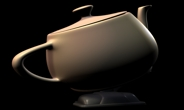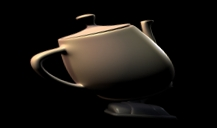matrix := list(1, 0.3, 0, 0.1, 1, 0, 0, 0, 1.0) result := i LinearTransform(matrix)
Translate(point, filterType)
point: Translate by a specified number of pixels (List)
filter: resampling filter to use (Number). Defaults to CatmullRom

Translate an image with appropriate resampling. Integral pixel translate operations are automatically optimized.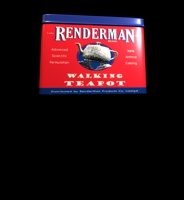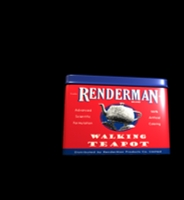filter := IceFilterType Bilinear amount := list(23.5. 60.6) result: = orig Translate(amount, filter)

This is a special case of LinearTransform.

Move(point)
point: Move by a specified number of pixels (List)

Returns a copy of the operand image with its world coordinates moved by an integral ammount. The copy will share data with the source, no resampling will be performed

Scale(scale, filter)
scale: Scale by specified factors in x and y (List)
filter: resampling filter to use. (Number) Defaults to CatmullRom

Geometrically scale an image with appropriate resampling.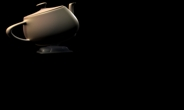filter := IceFilterType Lanczos amount := list(0.5. 0.5) result: = orig Scale(amount, filter)

This is a special case of LinearTransform.

Rotate(degrees, filter)
degrees: Angle of rotation in degrees (Number)
filter: resampling filter to use (Number). Defaults to CatmullRom

Rotate an image about (0, 0). The angle of rotation is measured counterclockwise.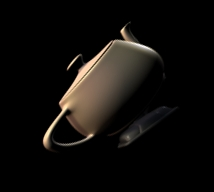This is a special case of LinearTransform.

Resize(scale)
scale: Scale by specified factors in x and y (List)

Fast geometric scaling using only point-sampling. Useful for previews and interactive display.

Reformat(newBox, preserveAR, crop, anamorph, filter)
newBox: Desired box (List)
preserveAR: Should the aspect ratio be preserved? (Boolean) default = true
crop: Should the image be cropped to preserve aspect ratio? (Boolean) default = true
anamorph: If the final image is destined for anamorphic display, the "stretch factor". (Number) default = 1.0
filter: resampling filter to use (Number). Defaults to CatmullRom

This is an operation that fulfills a common need: that of changing the size of an image prior to saving. The arguments are self-explanatory: letter- or window-boxing is automatically performed if aspect ratio is to be preserved and cropping is not enabled. The anamorph argument is unity when no anamorphic display is intended: larger than unity when it is.

Note: This is not a "native" operation: it internally comprises other operations arranged to yield the result desired.

Flip(x, y, transpose)
x: Boolean, flip in x?
y: Boolean, flip in y?
transpose: Boolean, transpose the image?

This operation is optimized to perform the eight possible "unity-scale" transformations. These are illustrated below.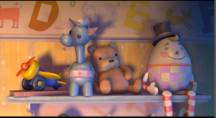# No-op result := m1_1 Flip(0, 0, 0)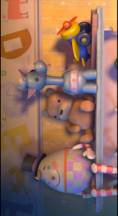# Transpose axes result := m1_1 Flip(0, 0, 1)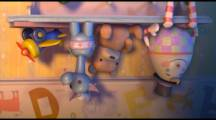# Flip in Y result := m1_1 Flip(0, 1, 0)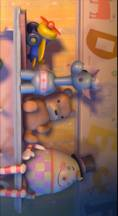# Flip in Y and transpose axes result := m1_1 Flip(0, 1, 1)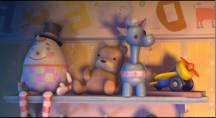# Flip in X result := m1_1 Flip(1, 0, 0)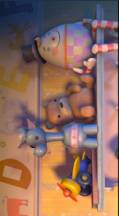# Flip in X and transpose axes result := m1_1 Flip(1, 0, 1)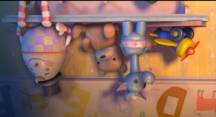# Flip in X and Y result := m1_1 Flip(1, 1, 0)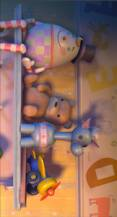# Flip in X and Y and transpose result := m1_1 Flip(1, 1, 1)
DisplacementWarp(warpImg, minMax, filterType, filterScale)
warpImg: Displacement image (Image)
minMax: Displacements corresponding to 0 and 1 (List) defaults to (0, 1)
filterType: Filter type. Defaults to CatmullRom
filterScale: Scale equivalent for filter width (Number) defaults to 1.0

General displacement warp operation. WarpImg is a two-channel vector field containing the offset of the source pixel for each result pixel. The actual offset is given by:

O' = o * (max − min) + min

The type of the filter is normally best set to FILTER_CATROM. Since it is not possible to analytically determine a single best filter width, filterScale should be chosen to yield the best possible combination of sharpness and anti-aliasing. 1.0 is a good place to start: smaller values yield wider filters (and less sharpness).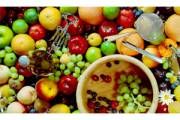Original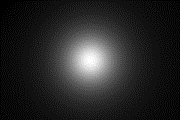Displacement Image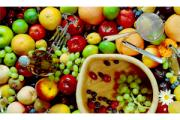Warped Image minMax := list (0, 10) filter := IceFilterType MitchellNetravali fScale := 1.0 result := fruit DisplacementWarp(disp, minMax, filter, fScale)